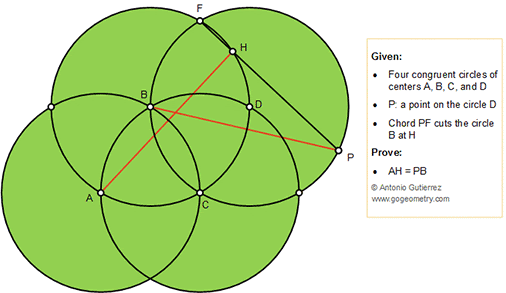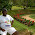## Thursday, December 6, 2018

### Geometry Problem 1405: Four Congruent Circles, Congruent Chords

Geometry Problem. Post your solution in the comment box below.
Level: Mathematics Education, High School, Honors Geometry, College.

Details: Click on the figure below.#### 3 comments:

1.From ang CFH and CFP => CH = CP
From congr of circles AC = BC
=> Tr ACH congr Tr BCP (SAS)

2.ABC, BCD, BDF are all equilateral triangles
ABF is a straight line and a diameter of circle B and so AH is perpendicular to FP

S(BFP) = 1/2.BP/2.FP......(1) (since < BPF = 30 since D is the centre of TR BFP)

S(AFP) = 1/2.AH. FP ......(2)

Since S(AFP) = 2.S(BFP) from (1) and (2) we have

AH = BP

Sumith Peiris
Moratuwa
Sri Lanka

3.CBF equal 120*. Angle CPF equal 60*. Angle CHP=FAC=CPE=60*.Triangle CHP equilateral. PC=PH=HC. R(C,60*) B-->A P-->H BP=AH. In triangle APH AP in second power=AH second power+ HC in second power=BP in second power + PC in second power. This means AP in second power=Bp in second power + PC in second power.

Erina,NJ